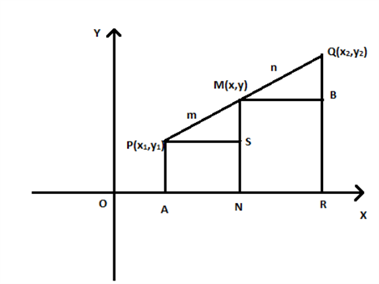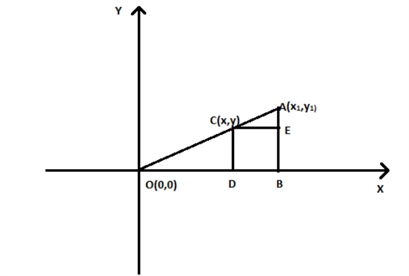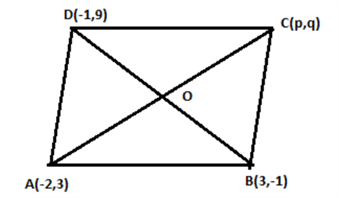# Section Formula & Conic Sections

A point on the line segment divides it into two parts which may equal or not. The ratio in which the point divides the given line segment can be found if we know the coordinates of that point. Also, it is possible to find the point of division if we know the ratio in which the line segment joining two points has given. These two things can be achieved with the help of a section formula in coordinate geometry.

Section formula is used to determine the coordinate of a point that divides a line segment joining two points into two parts such that the ratio of their length is m:n.

Let P and Q be the given two points (x1,y1) and (x2,y2) respectively, and M be the point dividing the line-segment PQ internally in the ratio m:n, then form the sectional formula for determining the coordinate of a point M is given by:

$$\begin{array}{l}\large M (x,y) = \left ( \frac{mx_{2}+nx_{1}}{m+n} , \frac{my_{2}+ny_{1}}{m+n} \right )\end{array}$$

## Proof for Sectional Formula

Let P(x1, y1) and Q(x2, y2) be two points in the xy – plane. Let M(x, y) be the point which divides line segment PQ internally in the ratio m : n.PA, MN and QR are drawn perpendicular to x- axis.

PS and MB are drawn parallel to x – axis.

∠MPS = ∠QMB (corresponding angles)

∠MSP = ∠QBM = 90°

By AA similarity criterion,

ΔPMS ~ ΔMQB

$$\begin{array}{l}\Rightarrow ~\frac{PM}{MQ} = \frac{PS}{MB} = \frac{MS}{QB} = \frac{m}{n} …(1)\end{array}$$

PS = AN = ON – OA = x – x1

MB = NR = OR – ON = x2 – x

MS = MN – SN = y – y1

QB = RQ – RB = y2 – y

From equation (1),

$$\begin{array}{l}\large \frac{m}{n} = \large \frac{x~-~x_1}{x_2~-~x} = \large \frac{y~-~y_1}{y_2~-~y}\end{array}$$
$$\begin{array}{l}\large ⇒~\frac{m}{n} = \large \frac{x~-~x_1}{x_2~-~x}\end{array}$$
$$\begin{array}{l}\large⇒~x = \large\frac{mx_2~+~nx_1}{m~+~n}\end{array}$$

Similarly,

$$\begin{array}{l}\large\frac{m}{n} = \large\frac{y~-~y_1}{y_2~-~y}\end{array}$$
$$\begin{array}{l}\large⇒~y = \large\frac{my_2~+~ny_1}{m~+~n}\end{array}$$

So, the coordinates of the point M(x,y) which divides the line segment joining points P(x1, y1) and Q (x2, y2) internally in the ratio m:n are

$$\begin{array}{l}\LARGE\left(\frac{mx_2~+~nx_1}{m~+~n},\frac{my_2~+~ny_1}{m~+~n}\right)\end{array}$$

This is known as section formula.

### Sectional formula (Externally):

Sectional Formula can also be used to find the coordinate of a point that lie outside the line, where the ratio of the length of a point from both the lines segments are in the ratio m:n.

The Sectional Formula is given as:

$$\begin{array}{l}\large \left ( \frac{mx_{2} – nx_{1}}{m – n} , \frac{my_{2} – ny_{1}}{m – n} \right )\end{array}$$

Let us understand it’s proof with an Example:

Consider a point A(x1, y1).

C(x, y) is a point which divides the line OA in the ratio 2 : 1, where point O is origin.

⇒ OC : CA = 2 : 1To find coordinates of the point C, three lines CD, AB and CE are drawn such that CD and AB are perpendicular to x – axis and CE is parallel to x – axis.

∠COD  =∠ACE (corresponding angles)

∠CDO = ∠AEC = 90°

By AA criterion for similarity of two triangles,

ΔOCD ~ΔCAE

So, the ratio of corresponding sides will be equal, i.e.

$$\begin{array}{l}\frac{OC}{CA} = \frac{CD}{AE} = \frac{OD}{CE}…(1)\end{array}$$

Since coordinates of C are (x, y),

OD = x units

BE = CD = y units

CE = BD = OB – OD = (x1 – x) units
AE = AB – BE = (y1 – y) units
Also, OC : CA = 2 : 1
⇒ OC = 2a units
And
CA = a units
Where a is a constant.

Equation(1) becomes

$$\begin{array}{l}\large \frac{2a}{a} = \large \frac{x}{x_1~-~x} = \large \frac{y}{y_1~-~y}\end{array}$$
$$\begin{array}{l}\large \frac{x}{x_1~-~x} = 2\end{array}$$
$$\begin{array}{l}\large ⇒~x = \large 2(x_1~-~x)\end{array}$$
$$\begin{array}{l}\large ⇒~3x = \large 2x_1\end{array}$$
$$\begin{array}{l}\large ⇒~x = \large \frac{2}{3}~x_1\end{array}$$

Similarly,

$$\begin{array}{l}\large \frac{y}{y_1~-~y} = \large 2\end{array}$$
$$\begin{array}{l}\large ⇒~3y = \large 2y_1\end{array}$$
$$\begin{array}{l}\large y = \large \frac{2}{3}~ y_1\end{array}$$

Therefore, the coordinates of the point C is

$$\begin{array}{l}(\large \frac{2}{3}~ x_1, \frac{2}{3}~ y_1)\end{array}$$

## Section Formula For Class 10

The coordinates of the point A(x, y) which divides the line segment joining the points P(x1, y1) and Q(x2, y2) internally in the ratio m1 : m2 are given by the formula:

$$\begin{array}{l}P(x, y) = \large \left ( \frac{m_{1}x_{2} + m_{2}x_{1}}{m_{1} + m_{2}} , \frac{m_{1}y_{2} + m_{2}y_{1}}{m_{1} + m_{2}} \right )\end{array}$$

Similarly, if the point P divides the line joining A and B externally in the ratio m1 : m2, then the coordinates of the points are:

$$\begin{array}{l}P(x, y) = \large \left ( \frac{m_{1}x_{2} – m_{2}x_{1}}{m_{1} – m_{2}} , \frac{m_{1}y_{2} – m_{2}y_{1}}{m_{1} – m_{2}} \right )\end{array}$$

If the point M divides the line segment joining points P(x1, y1) and Q(x2, y2)  internally in the ratio k : 1, then coordinates of  M will be:

$$\begin{array}{l}M(x, y) = \large \left(\frac{kx_2~+~x_1}{k~+~1},\frac{ky_2~+~y_1}{k~+~1}\right)\end{array}$$

Similarly,  the formula for external division is:

$$\begin{array}{l}M(x, y) = \large \left(\frac{kx_2~-~x_1}{k~-~1},\frac{ky_2~-~y_1}{k~-~1}\right)\end{array}$$

Special Case:

What if the point M which divides the line segment joining points P(x1, y1) and Q(x2, y2) is midpoint of line segment PQ?

If M is the midpoint, then M divides the line segment PQ in the ratio 1:1, i.e. m=n=1.

Coordinates of point M are

$$\begin{array}{l}\large \left(\frac{1~×~x_2~+~1~×~x_1}{1~+~1}, \frac{1~×~y_2~+~1~×~y_1}{1~+~1}\right)\end{array}$$

Therefore, coordinates of a point which is the midpoint of line segment joining points P(x1, y1) and Q(x2, y2) are,

$$\begin{array}{l}\large \left(\frac{x_1~+~x_2}{2}, \frac{y_1~+~y_2}{2}\right)\end{array}$$

## Video Lessons

### Section Formula – Introduction## Section Formula Examples

Example 1: Find the coordinates of the point which divides the line segment joining the points (4,6) and (-5,-4) internally in the ratio 3:2.

Solution: Let P(x, y) be the point which divides the line segment joining A(4, 6) and B(-5, -4) internally in the ratio 3 : 2.

Here,

(x1, y1) = (4, 6)

(x2, y2) = (-5, -4)

m : n = 3 : 2

Using the section formula,

$$\begin{array}{l}P(x, y) = \large \left(\frac{mx_2~+~nx_1}{m~+~n}, \frac{my_2~+~ny_1}{m~+~n}\right)\end{array}$$

Coordinates of P are,

$$\begin{array}{l}x=\large\frac{3~×~-~5~+~2~×~4}{3~+~2}\end{array}$$
$$\begin{array}{l}y=\large \frac{3×~-~4~+~2~×~6}{3~+~2}\end{array}$$
$$\begin{array}{l}\Rightarrow~x = \large \frac{-15~+~8}{5}\end{array}$$
$$\begin{array}{l}\Rightarrow~y = \large \frac{-12~+~12}{5}\end{array}$$
$$\begin{array}{l}\Rightarrow~x= \large -\frac{7}{5}\end{array}$$
$$\begin{array}{l}\Rightarrow~y = 0\end{array}$$

Example 2: The 4 vertices of a parallelogram are A(-2, 3), B(3, -1), C(p, q) and D(-1, 9). Find the value of  p and q.

Solution: Given vertices of a parallelogram are:

A(-2, 3), B(3, -1), C(p, q) and D(-1, 9)

We know that diagonals of a parallelogram bisect each other.Let O be the point at which diagonals intersect.

$$\begin{array}{l}\text{Coordinates of mid-points of both } \overleftrightarrow{AC} \text{ and } \overleftrightarrow{BD} \text{will be same.}\end{array}$$

Therefore,

Using midpoint section formula,

$$\begin{array}{l}\large \left(\frac{x_1~+~x_2}{2}, \frac{y_1~+~y_2}{2}\right)\end{array}$$
$$\begin{array}{l}\large \frac{-2~+~p}{2} = \large \frac{-1+3}{2}\end{array}$$
$$\begin{array}{l}\large \Rightarrow ~-2~+~p =2\end{array}$$

p = 2 + 2

p = 4

Similarly,

$$\begin{array}{l}\large\frac{3~+~q}{2} =\frac{9~-~1}{2}\end{array}$$
$$\begin{array}{l}3~+~q = 8\end{array}$$

q = 8 – 3

q = 5